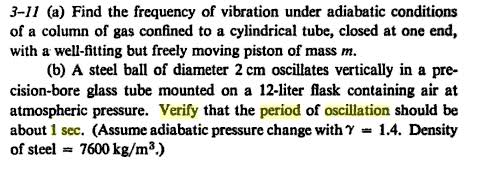# Period of Oscillation of Steel Ball Help Please!I already did the first part, and the equation for the period becomes: T=2$$\pi$$(ml/$$\gamma$$PA)(1/2)

I know 12 litres = 0.012 m3, and the the mass of the ball is the volume of a sphere of radius 0.01m x the density = 0.0318 Kg, for pressure I am not sure whether I am suppose to use 1 atm or 101.3 kPa, that's the least of my problems though. The area of the a cross section of the tube, assuming the ball is fitted, is $$\pi$$(0.01)2=0.000314m3...So assuming all those are right, now I need the length. I thought that maybe the flask also had the same radius as the ball, and so the length would just be 0.012m3/0.0000314m3=38.2m, but when I plug all there numbers in to the equation for period I don't get 1 second or anything close as the answer.

Edit: Hm...if I use 101 325 Pa as my pressure it works....can someone confirm if what I've done is right? 38.2 m seems awfully long for a flask.

Last edited: